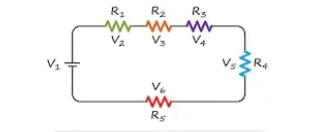### Ticker

6/recent/ticker-posts

# DC BASIC AND NETWORK PART:- 2 (KCL & kVL Law)

KIRCHHOFF'S LAWS

Gustov Robert kirchhoff derived the laws known kirchhoff's laws which are nothing but extension of OHM'S LawKCL & kVL law

1. Kirchhoff's First law
Current law ( KCL ) or Point law

According to this law in any Network of wires carrying Current, the algebraic sum of all current meeting at a point or juction is zero or the same of incoming currents towards any point is equal to the same of outgoing current away From that point. If I1, I2, I3, I4, I5 and I6 are the current meeting at juction O flowing in the direction of Arrowhead marked on them fig. Taking incoming currents as possitive and outgoing current is nagative, according to kirchhoff's First law.

Example :-

I1 - I2 = 0,  I1  =  I2

Incoming current( I1 )

------------->-----------•O---------->-------------
Positive (+)                     Outgoing
Current ( I2 )
Nagative (-)KCL law

I1 + I2 + I3 - I4 -I5   = 0

I4 + I5  = I1 + I2 + I3

Kirchhoff's Second law or Voltage law( KVL ) or Mesh law

According to this law in any closed Circuit or mesh the algebraic sum of emfs acting in that Circuit or mesh is equal to the algebraic sum of the products of the currents and resistance of each part of the Circuit.
If the Circuit shown in fig. Is considered, then according to kirchhoff's second law or ( kVL).

Only For Understood Here ---> in fig.

I2   + E2 -     R3          R4
E|---<---||-----^^^^^-----^^^^^-------|D
I2 v                                             v I2
|             R1     I1 + I2     R2   |
F |--------^^^^^^---->----^^^^^^----•|C
I1 ^                                              v I1
|     +E1-                    R5        |
|--<--||--------------^^^^^^^---------|
A      I1                                       B

In mesh  AFCBA

E1 = R1 ( I1 + I2 ) + R2( I1 + I2 ) + R5 I1
OR
E1 = ( R1+ R2 )  ( I1 + I2 ) + R5 I1

In Mesh FEDCF

- E2 = - R3 I2 - R4 I2 - R2 ( I1 + I2 )
- R1 ( I1 + I2 )
OR
E2 = R3 I2 + R4 I2 +( R1 + R2 ) ( I1 + I2 )

And in Mesh AFEDCBA,

E1 - E2 = - R3 I2 - R4 I2 + R5 I1
OR
E1 - E2 = R5 I1 - ( R3 + R4 ) I2

Here is simple law of KVL only for UnderstoodkVL lawV1 + V2 + V3 + V4 + V5 + V6 = 0

Voltage source direction positive (+) site
Means

---->   + Battery  <---        R (load)
<---
V   ---||---------------^^^^^^-------

Because Voltage source and voltage drop on the load algebraic sume = 0

Voltage source = Voltage Drop
Voltage source + voltage drop = 0

Voltage source (+) Site ----> Coming in possitive terminal site (-v)

Voltage source(-) ----->
And out  of possitive terminal site  (+v)

Voltage Source direction nagative (-) site